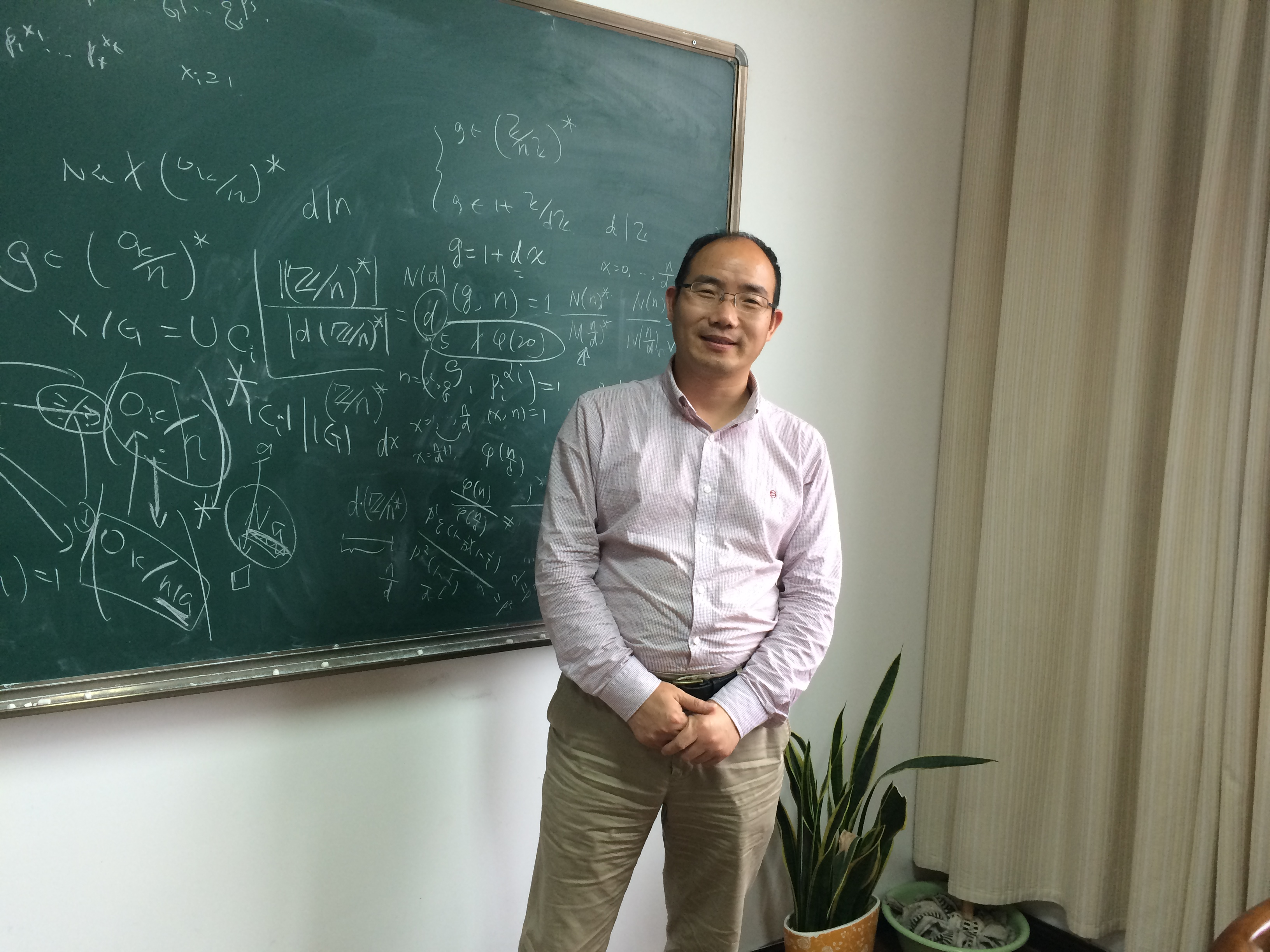# Chungang Ji ProfessorBio:

### Educational Background:

• PhD, Pure Mathematics, Tongji University (2001)
• MA, Pure Mathematics, University of Science and Technology of China (1993)
• BA, Mathematical Education, Nanjing Normal University (1990)

### Research

Research Interests

Algebraic number theory, Arithmetic algebraic geometry.

Research Projects:

(1) Quadratic forms over the rings of algebraic integers, K-theory and beyond (Grant No.11471162), Chungang Ji, NNSF of China, from 2015-01 to 2018-12.

(2) Sums of squares in the rings of integers of biquadratic number fields and beyond (Grant No.20133207110012), Chungang Ji, SRFDP of Higher Education of China, from 2014-01 to 2016-12.

(3) L-functions, quadratic fields and beyond (Grant No.10971098), Chungang Ji, NNSF of China, from 2010-01 to 2012-12.

(4) Special value of L-functions (Grant No.10201013), Chungang Ji, NNSF of China, from 2003-01 to 2005-12.

(5) Chinese Postdoctoral Science Foundation, 32th, Chungang Ji, from 2002-02 to 2004-01.

(6) Postdoctoral Science Foundation of Jiangsu Province, 2nd, Class B, Chungang Ji, from 2005-05 to 2007-04.

Journal Articles(Selected)

(1) Bin Zhang; Chungang Ji. Sums of three integral squares in biquadratic fields.  J. Number Theory 138(2014), 37–47.

(2) ChunGang Ji. A specific family of cyclotomic polynomials of order three. Sci. China Math. 53(2010), no. 9, 2269–2274.

(3) Qingzhong Ji; Chungang Ji. On the periodicity of some Farhi arithmetical functions. Proc. Amer. Math. Soc. 138(2010), no. 9, 3025–3035.

(4) Chungang Ji; Weiping Li; Pieter Moree. Values of coefficients of cyclotomic polynomials. II. Discrete Math. 309(2009), no. 6, 1720–1723.

(5) Chungang Ji; Weiping Li. Values of coefficients of cyclotomic polynomials.  Discrete Math. 308(2008), no. 23, 5860–5863.

(6) Chungang Ji; Yan Xue. An elementary proof of the law of quadratic reciprocity over function fields. Proc. Amer. Math. Soc. 136(2008), no. 9, 3035–3039.

(7) Dasheng Wei; Chungang Ji. On the number of certain Galois extensions of local fields. Proc. Amer. Math. Soc. 135(2007), no. 10, 3041–3047.

(8) Chungang Ji; Dasheng Wei. Sums of integral squares in cyclotomic fields. C. R. Math. Acad. Sci. Paris 344(2007), no. 7, 413–416.

(9) Chungang Ji; Yuanhua Wang; Fei Xu. Sums of three squares over imaginary quadratic fields. Forum Math.18(2006), no. 4, 585–601.

(10) Chungang Ji. A simple proof of a curious congruence by Zhao. Proc. Amer. Math. Soc. 133(2005), no. 12,3469–3472.

(11) Chungang Ji. Diophantine equations x^2+Dy^2=-1, ±2, odd graphs, and their applications. J. Number Theory 114(2005), no. 1, 18–36.

(12) Chungang Ji; Hongwen Lu. Lower bound of real primitive L-function at s=1. Acta Arith. 111(2004), no. 4, 405–409.

(13) Chungang Ji. Sums of three integral squares in cyclotomic fields. Bull. Austral. Math. Soc. 68(2003), no. 1, 101–106.

(14) Hongwen Lu; Rongzheng Jiao; Chungang Ji. Dedekind zeta-functions and Dedekind sums. Sci. China Ser. A 45(2002), no. 8, 1059–1065.

(15) Yonggao Chen; Chungang Ji. On a function related to multinomial coefficients. I. Acta Math. Sin. (Engl. Ser.) 18(2002), no. 4, 647–660.

(16) Peter Bundschuh; Chungang Ji; Zun Shan. A remarkable class of congruences. Acta Sci. Math. (Szeged) 67(2001), no. 3-4, 493–500.

(17) Hongwen Lu; Chungang Ji; Rongzheng Jiao. Kronecker limit formula for real quadratic number fields. III. Sci. China Ser. A 44 (2001), no. 9, 1132–1138.

(18) Yonggao Chen; Chungang Ji. The number of multinomial coefficients not divided by a prime. Acta Sci. Math. (Szeged) 64(1998), no. 1-2, 37–48.

### Honors and Awards

The 2nd Mathematical Achievement Award of Jiangsu Province(2010)

(1) The reviewer of Mathematical Reviews(Since 2005);

(2) The reviewer of Zentralblatt MATH(Since 2007).